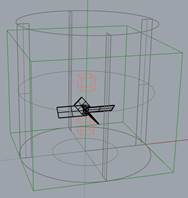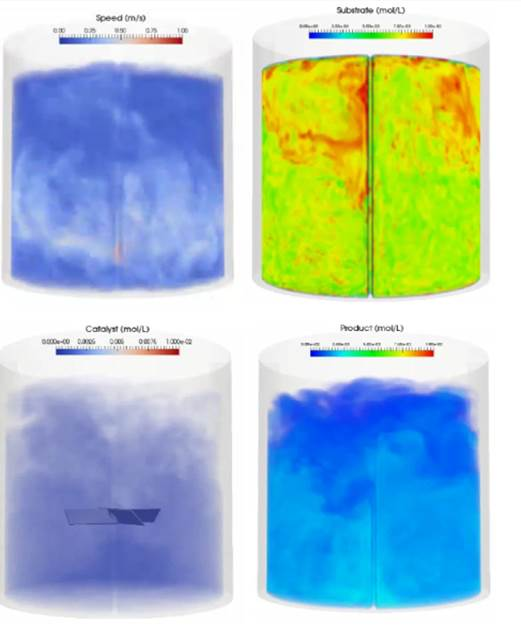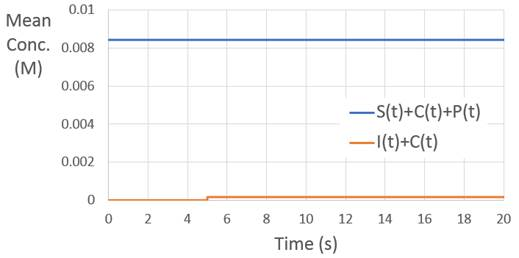# Modeling Chemical Reactions¶Simulation Overview

• Model a chemical reaction in M-Star CFD

• Predict time evolution of species production

• Assess if process is reaction limited

• Suggest improvements for decreasing processing time

• 0.3 m tall x 0.3 m diameter dish bottomed baffled tank

• 0.125 m pitch blade impeller,

• Impeller centered in the tank and 0.096 m off-bottom

• 180 RPM impeller rotation rate

• Water at STP

• Modeling two-step catalyst assisted reaction

$\begin{split}S + C \mathrel{\substack{k_1\\\rightleftarrows\\ k_{-1}}} I \overset{k_2}{\rightarrow} P + C\end{split}$

Governing Equations

$\begin{split}\frac{dS}{dt} &= k_{-1} I - k_1 S C \\ \frac{dC}{dt} &= (k_{-1} + k_2)I - k_1 S C \\ \frac{dI}{dt} &= k_1 S C - (k_{-1} + k_2) I \\ \frac{dP}{dt} &= k_2 I \\ \text{where,} \\ k_1 &= 500 M^{-1} S^{-1} \\ k_{-1} &= 10 M^{-1} S^{-1} \\ k_2 &= 100 M^{-1} S^{-1}\end{split}$
• Substrate (reversibly) reacts with catalyst to form intermediate

• Intermediate breaks down to form product and recover catalyst

• Expect to satisfy conservation laws:

$\begin{split}I + C &= I_0 \\ S + C + P &= S_0\end{split}$

Modeling Approach

We choose to define four scalar fields here: S, C, I, P. The initial substrate, S, is injected into the system at time zero via a scalar injection domain. The catalyst, C, is injected into the system five seconds later into a second injection domain. The initial concentrations of scalar fields, I and P are initially set to zero and no injection domains are defined. Instead, these species will be produced throughout the tank via the chemical reaction between S and C. The input to each scalar field is summarized below.

 Scalar Name S C I P Diffusion Coefficient 1.00E-09 1.00E-09 1.00E-09 1.00E-09 Reaction Chemistry 10*I-500*S*C (10+100)*I-500*S*C 500*S*C - (10+100)*I 100*I Starting Concentration 0 0 0 0 Box Lower Corner -0.0145, 0.145, -0.0145 -0.0145, 0.045, -0.0145 0,0,0 0,0,0 Box Upper Corner 0.0145, 0.174, 0.0145 0.0145, 0.074, 0.0145 0,0,0 0,0,0 Box Starting Concentration 5 0.1 0 0 Start Time 0 5 0 0 Stop Time 0 0 0 0
• System resolution: 150 lattice points (dx=0.0019 m)

• Set the output intervals - DataOutputInterval, SliceOutputInterval, and volumeOutputInterval: 0.005 s, 0.05 s, 0.1 s

Data Collection

The data output can be visualized in ParaView using the MStarPost3D files, colored according to species concentration. The output at one snap shot is illustrated below. Movies depicting the time-evolution of the fluid flow and species transport/reactions are available here:Fig. 7 Speed and Species Concentrations at t=7 seconds

In addition to the visual output, the solver automatically produces a SpeciesData_N.dat file for each system, from which we obtain the time-evolution of the mean concentration of each species. These results are summarized below.Fig. 8 Time-evolution of species concentrations

For times less than 5 seconds, the mean concentration of the substrate is constant. Although the species is becoming increasingly homogenized, the system is closed and the total concentration is not changing. After five seconds, the catalyst is added to the system. This catalyst motivates the production of the product. At short times, the production of the product is not uniform. At long times, as the catalyst becomes homogenized, production becomes uniform. Note that the decrease/increase in substrate/product concentration with time is due to the chemical reaction, not an opening in the system.

The numerical veracity of the reactions can be validated by examining species conservation laws. Across all times, the sum of the substrate concentration, catalyst concentration, and product concentration remains constant. Likewise, following the injection of the catalyst, the sum of the catalyst concentration and intermediate species concentration remains constant.Fig. 9 Species conservation laws

Data Analysis/Results.

At short times, product is produced non-uniformly through the tank as the catalyst moves under the action of the impeller. Indeed, for the first few seconds following catalyst injection, a reaction front can be observed at boundary of the catalyst. For this short period, the process is pumping limited, not reaction limited. After a few seconds, however, the catalyst becomes homogenized and the product is produced nearly uniformly through the tank. At this point, increasing the agitator speed has little to no effect on reaction rates; the process is reaction limited, not mixing limited. As such, modifications to stoichiometry (e.g. more catalyst to increase reaction rage) will result in faster processing than modification to impeller speed or configuration.